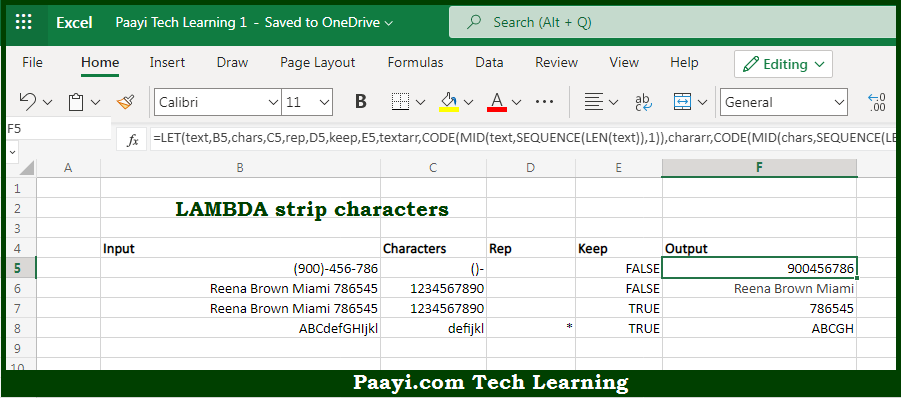# Learn How to LAMBDA Strip Characters in Microsoft Excel

Written by | 0 Comments | 532 Views

In this article, you will learn how to evaluate things in Dynamic Arrays with formulas in Microsoft Excel using a single/combination(s) of functions. You will also know How to LAMBDA Strip Characters and see the generic formula.

Learn How to LAMBDA Strip Characters in Microsoft Excel

The main purpose of this formula is to strip characters from text. Here we will learn how to strip characters in the given data range in the workbook in Microsoft Excel. That implies, with the help of a formula based on the LAMBDA function you can able to strip characters from text. So, with the help of this formula, you can able to strip characters in the given data range in the workbook in Microsoft Excel.

General Formula to LAMBDA Strip Characters

=LET(text,B5,chars,C5,rep,D5,keep,E5,textarr,CODE(MID(text,SEQUENCE(LEN(text)),1)),

chararr,CODE(MID(chars,SEQUENCE(LEN(chars)),1)),TEXTJOIN("",1,IF(ABS(keep-ISNUMBER(MATCH(textarr,chararr,0))),rep&"",CHAR(textarr))))

The Explanation for LAMBDA Strip CharactersSo we know that with the help of the given formula above you can able to strip characters from text. Here we will learn how to strip characters in the given data range in the workbook in Microsoft Excel. As we know that the first step in creating a custom LAMBDA function is to verify the logic required in the standard formula.

The formula is having four inputs:

text - the incoming text
chars - the characters to strip
rep - the character to replace stripped characters with
keep - strip or preserve chars, where FALSE = strip, TRUE = preserve

The keep parameter is a boolean that "flips" the behavior of the function from tripping characters to preserving characters. So, with the help of this formula, you can able to strip characters from text. Here we will learn how to strip characters in the given data range in the workbook in Microsoft Excel.